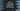# Python string upper() method explanation with example## Python string upper() method explanation with example:

Python string upper() method is used to convert each character of the string to upper case. This method is defined as below :

``string.upper()``

This method doesn’t take any parameter and it returns the new string with all characters as uppercase.

### Example of python upper:

Let me show you an example of python upper:

``````given_string = "Hello World !!"

print(given_string.upper())``````

This program will print:

``HELLO WORLD !!``

### Use python upper by taking user input:

Let’s change the above program to take one string as input from the user and convert it to uppercase:

``````given_string = input("Enter a string : ")

print("Lowercase : {}".format(given_string.upper()))``````

It will give output as like below:

``````Enter a string : Hello World !!
Lowercase : HELLO WORLD !!``````

### Example to compare two strings by converting strings to upper:

We can use upper() to convert a string to upper case and convert it with a different string by converting that string to upper. For example:

``````first_string = input("Enter a string : ")
second_string = input("Enter the second string : ")

if(first_string.upper() == second_string.upper()):
print("Both strings are equal")
else:
print("Strings are not equal")``````

In this program, we are comparing two strings without considering the cases.

It will print outputs like below:

``````Enter a string : hello world
Enter the second string : Hello World
Both strings are equal

Enter a string : hello
Enter the second string : world
Strings are not equal``````